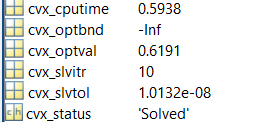# LASSO Problem with CVX and ADMM

I am solving the Lasso problem
minimize 1/2*|| Ax - b ||_2^2 + lambda || x ||_1
with CVX and getting good results for my study but when I apply ADMM algorithm I am getting very different results. Why is there such a difference? I would like to understand the solution of the problem better but cant follow what CVX does.

Is the optimal objective value the same, within a reasonable tolerance? If so, perhaps the argnin is no unique. Does CVX report the problem is solved to optimality? How about ADMM? You have not shown us any code or any example or results, so how can we provide reasonable explanations?

A=[60,60] b=[60,400] complex valued matrices.
using the code below

for i=1:length(b(1,:))
cvx_begin quiet
variable x(a)
minimize(1e-3norm(x,1)+(norm(Ax-b(:,i),2)))
cvx_end
gg(:,i)=x;
end
and getting thison the other hand for ADMM I am using the function in here https://web.stanford.edu/~boyd/papers/admm/lasso/lasso.html

for i=1:length(b(1,:))

% lambda_max = norm( A’*b(:,i), ‘inf’ );
lambda = 1e-3;
[x_0 history] = lasso(A, b(:,i), lambda, 1, 1);
gg(:,i)=x_0;
end

and in history.objval have 1000 values they are going like this

and end up being NAN.

Well, then you have a (serious, diverging) problem with the performance of the implemented ADMM algorithm, which is out of scope of this forum.

Okay then is it possible to see what steps/methods are applied in CVX to solve the problem. And maybe implement it by myself?

I believe CVX transforms a LASSO problem into an SOCP, then calls an SOCP capable solver to solve it.